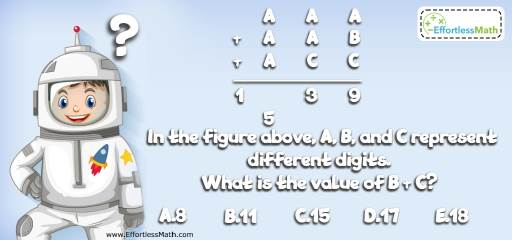# Intelligent Math Puzzle – Challenge 89

This mind-blowing mathematics and critical thinking challenge is not for the faint at heart—or mind. Can you solve it?## Challenge:

In the figure above, A, B, and C represent different digits. What is the value of B + C?

A- 8

B- 11

C- 15

D- 17

E- 18

### The Absolute Best Book to Challenge Your Smart Student!

In this problem, we can write a system of three equations and three variables.
Let’s review the equations.
A + B + C = 9 or 19
A + A + C = 5 or 15
A + A + A = 13 or 12
3A cannot be 13. Therefore, 3A = 12 ⇒ A = 4
We can also conclude that in the second equation:
A + A + C = 15 ⇒ 2A + C = 15 ⇒ C = 7
Or A + A + C = 16 (1 may added from the first column)
C = 6
Try different values for the first equation:
A + B + C = 9 or 19
If A = 4 and C = 6 ⇒ B = 9
If A = 4 and C = 7 ⇒ B = 8
B + C = 15

The Best Books to Ace Algebra

### What people say about "Intelligent Math Puzzle – Challenge 89 - Effortless Math: We Help Students Learn to LOVE Mathematics"?

No one replied yet.

X
45% OFF

Limited time only!

Save Over 45%

SAVE $40 It was$89.99 now it is \$49.99